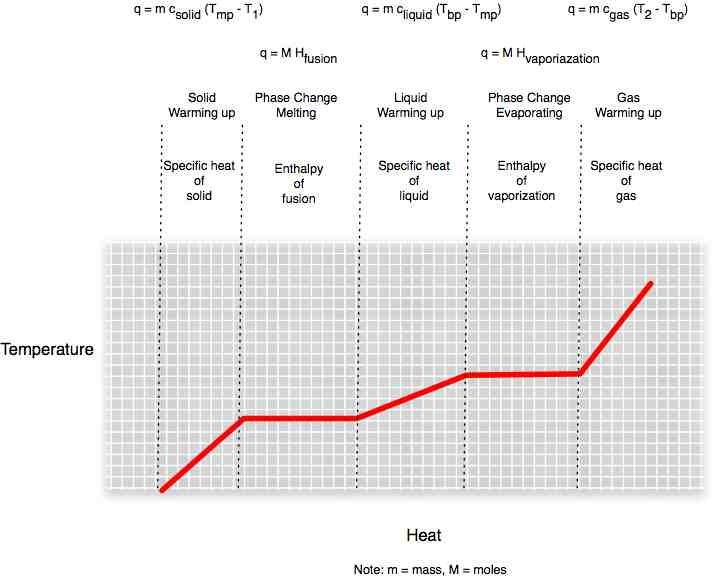# Chemistry

#### Chemistry 12: 4-7WebLecture

# Other Kinds of Solid States

## Energy Considerations

As with liquids, the forces holding the ionic pairs together will determine the work needed to pull the lattice apart, or dissolve it in solution.

#### Lattice Energy

Lattice energy is the energy required to break up an ionic solid. This is tricker than determining the energy required to break a bond between two atoms in a covalent molecular structure, since an ion usually is susceptible to forces from multiple charges.

The formula for lattice energy is related to the formula for force between two charged particles, because work is the result of a force applied to move some mass over a distance d: U = F * d.

The force equation between two electrical charges is Fe = kQq/d2.
The energy equation is U = CNA [(n+e)(n-e)/d].
The total positive charge Q = n+e.
The total negative charge q = n-e.
The constant k = CNA.

#### Forms of Solids

Molecules form solids when they are held together by van der Waals forces (those transient dispersion forces from chapter 12). These can form lattices as well, but the units are the entire molecule. Network solids and amorphous solids are formed of individual atoms that share covalent bonds with multiple atoms. In network solids, the bonds of regular, but in amorphous solids, the bonds are of different lengths. This gives amorphous solids the interesting property of having a temperature range for the melting and boiling points.

Take a look at these two forms of the same substance -- Silicon dioxide (SiO2).Quartz is a network solid with a fixed structure. Glass is an amorphous solid with the same molecules in rings and changes that have irregular structures. The amorphous nature of glass gives it a long melting range, which makes it possible to work and blow glass into fantastic shapes.

### Phase Changes

We can plot the amount of heat we pour into a substance. As we add heat energy to a solid, temperature rises until we reach the melting pot. Temperature does not rise while the heat energy is being used to break whatever bonds hold the molecules or atoms of the substance together. Once in liquid state, heat energy is used to raise the temperature until we reach the boiling point. As energy goes into freeing atoms from even the small bonds that join liquid molecules, the temperature stabilizes again. Once in gas form, temperature rises again as we add heat.Another common way of representing phase changes is the phase diagram. We map the point at which the substance turns from solid to liquid as pressure and temperature increase. At the triple point, the substance can move easily from solid to liquid, liquid to gas, or solid to gas, and back. The triple point for water is just above the freezing point. At temperatures above the triple point, but pressures below the critical pressure, the line between liquid and vapor slopes upward. Above the critical temperature, the substance cannot exist as a normal liquid or solid. Depending on the pressure, it is either a gas or a supercritical fluid.### Practice with the Concepts

#### Phases of Matter

At temperatures above the critical point, how does a substance behave?

### Discussion Questions

• How is the unit cell structure of an ionic solid determined?
• Can an ionic solid have more than one unit cell configuration?